### Quadratic Equations Class 10th Mathematics CBSE Solution

##### Question 1.Find the nature of the roots of the following quadratic equations. If the real roots exist, find them:(i) 2x2 – 3x + 5 = 0(ii)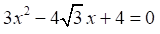(iii) 2x2 – 6x + 3 = 0Answer:There are three types of roots that are possible for a quadratic equation:For a quadratic equation: a x2 + b x + c = 0, we know that its roots is given by the formula(i) Comparing this equation with ax2 + bx + c = 0, we obtain,a = 2, b = −3, c = 5Discriminant D = b2 − 4ac = (− 3)2 − 4 (2) (5) = 9 − 40 = −31As, b2 − 4ac < 0,Therefore, no real root is possible for the given equation.(ii) Comparing this equation with ax2 + bx + c = 0,we obtain,a = 3b = -4√3c = 4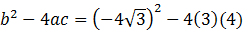Discriminant = 48 − 48 = 0As, b2 − 4ac = 0,Therefore, real roots exist for the given equation and they are equal to each other.And the roots will be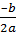and.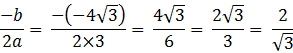Therefore, the roots are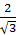and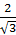.(iii) Comparing this equation with ax2+bx + c = 0,we obtain,a = 2, b = −6, c = 3Discriminant = b2 − 4ac = (− 6)2 − 4 (2) (3) = 36 − 24 = 12As, b2 − 4ac > 0,Therefore, distinct real roots exist for this equation as follows.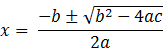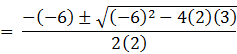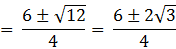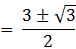So, the roots are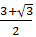or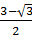.Question 2.Find the values of k for each of the following quadratic equations, so that they have two equal roots.(i) 2x2+ kx + 3 = 0(ii) kx (x – 2) + 6 = 0Answer:We know that if an equation ax2 + bx + c = 0 has two equal roots,its discriminant(b2 − 4ac) will be 0.(i) 2x2 + kx + 3 = 0Comparing equation with ax2 + bx + c = 0, we obtain,a = 2, b = k, c = 3Discriminate = b2 − 4ac = (k)2− 4(2) (3) = k2 − 24For equal roots,Discriminant = 0k2 − 24 = 0k2 = 24=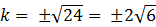(ii) kx (x − 2) + 6 = 0or kx2− 2kx + 6 = 0Comparing this equation with ax2 + bx + c = 0, we obtain,a = k, b = −2 k, c = 6Discriminant = b2 − 4ac = (− 2k)2 − 4 (k) (6) = 4k2 − 24kFor equal roots, b2 − 4ac = 0= 4k2 − 24k = 0= 4k (k − 6) = 0Either 4k = 0 or k = 6= k = 0 or k = 6However, if k = 0, then the equation will not have the terms ‘x2’ and ‘x’.Therefore, if this equation has two equal roots, k should be 6 only.Question 3.Is it possible to design a rectangular mango grove whose length is twice its breadth, and the area is 800 m2? If so, find its length and breadth.Answer:Let the breadth of mango grove be l.Length of mango grove will be 2l.Area of mango grove = (2l) (l) = 2l22l2 = 800=⇒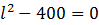⇒ l2 = 400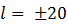However, length cannot be negative.Therefore, breadth of mango grove = 20 mLength of mango grove = 2 × 20 = 40 mQuestion 4.Is the following situation possible? If so, determine their present ages.The sum of the ages of two friends is 20 years. Four years ago, the product of their ages in years was 48.Answer:Let the age of one friend be x years.Age of the other friend will be (20 − x) years.4 years ago,age of 1st friend = (x − 4) yearsAnd, age of 2nd friend = (20 − x − 4) = (16 − x) yearsGiven that,(x − 4) (16 − x) = 4816x − 64 − x2 + 4x = 48x2 − 20x + 112 = 0Comparing this equation with ax2 + bx + c = 0, we obtaina = 1, b = −20, c = 112Discriminant = b2 − 4ac = (− 20)2 − 4 (1) (112) = 400 − 448 = −48As b2 − 4ac < 0,Therefore, no real root is possible for this equation and hence, this situation is not possible.Question 5.Is it possible to design a rectangular park of perimeter 80 m and area 400 m2? If so, find its length and breadth.Answer:Let the length and breadth of the park be l and b.Perimeter = 2 (l + b) = 80l + b = 40 Or, b = 40 – lArea = l × b = l (40 − l)= 40l − l2= 400 Givenl2 − 40l + 400 = 0Comparing this equation with al2 + bl + c = 0, we obtaina = 1, b = −40, c = 400Discriminant D = b2 − 4ac = (− 40)2 −4 (1) (400) = 1600 − 1600 = 0As b2 − 4ac = 0,Therefore, this equation has equal real roots and hence, this situation is possible.Root of this equation,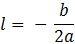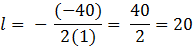Therefore, length of park, l = 20 mAnd breadth of park, b = 40 − l = 40 − 20 = 20 m

PDF FILE TO YOUR EMAIL IMMEDIATELY PURCHASE NOTES & PAPER SOLUTION. @ Rs. 50/- each (GST extra)

HINDI ENTIRE PAPER SOLUTION

MARATHI PAPER SOLUTION

SSC MATHS I PAPER SOLUTION

SSC MATHS II PAPER SOLUTION

SSC SCIENCE I PAPER SOLUTION

SSC SCIENCE II PAPER SOLUTION

SSC ENGLISH PAPER SOLUTION

SSC & HSC ENGLISH WRITING SKILL

HSC ACCOUNTS NOTES

HSC OCM NOTES

HSC ECONOMICS NOTES

HSC SECRETARIAL PRACTICE NOTES

# 2019 Board Paper Solution

HSC ENGLISH SET A 2019 21st February, 2019

HSC ENGLISH SET B 2019 21st February, 2019

HSC ENGLISH SET C 2019 21st February, 2019

HSC ENGLISH SET D 2019 21st February, 2019

SECRETARIAL PRACTICE (S.P) 2019 25th February, 2019

HSC XII PHYSICS 2019 25th February, 2019

CHEMISTRY XII HSC SOLUTION 27th, February, 2019

OCM PAPER SOLUTION 2019 27th, February, 2019

HSC MATHS PAPER SOLUTION COMMERCE, 2nd March, 2019

HSC MATHS PAPER SOLUTION SCIENCE 2nd, March, 2019

SSC ENGLISH STD 10 5TH MARCH, 2019.

HSC XII ACCOUNTS 2019 6th March, 2019

HSC XII BIOLOGY 2019 6TH March, 2019

HSC XII ECONOMICS 9Th March 2019

SSC Maths I March 2019 Solution 10th Standard11th, March, 2019

SSC MATHS II MARCH 2019 SOLUTION 10TH STD.13th March, 2019

SSC SCIENCE I MARCH 2019 SOLUTION 10TH STD. 15th March, 2019.

SSC SCIENCE II MARCH 2019 SOLUTION 10TH STD. 18th March, 2019.

SSC SOCIAL SCIENCE I MARCH 2019 SOLUTION20th March, 2019

SSC SOCIAL SCIENCE II MARCH 2019 SOLUTION, 22nd March, 2019

XII CBSE - BOARD - MARCH - 2019 ENGLISH - QP + SOLUTIONS, 2nd March, 2019

# HSCMaharashtraBoardPapers2020

(Std 12th English Medium)

HSC ECONOMICS MARCH 2020

HSC OCM MARCH 2020

HSC ACCOUNTS MARCH 2020

HSC S.P. MARCH 2020

HSC ENGLISH MARCH 2020

HSC HINDI MARCH 2020

HSC MARATHI MARCH 2020

HSC MATHS MARCH 2020

# SSCMaharashtraBoardPapers2020

(Std 10th English Medium)

English MARCH 2020

HindI MARCH 2020

Hindi (Composite) MARCH 2020

Marathi MARCH 2020

Mathematics (Paper 1) MARCH 2020

Mathematics (Paper 2) MARCH 2020

Sanskrit MARCH 2020

Sanskrit (Composite) MARCH 2020

Science (Paper 1) MARCH 2020

Science (Paper 2)

Geography Model Set 1 2020-2021

MUST REMEMBER THINGS on the day of Exam

Are you prepared? for English Grammar in Board Exam.

Paper Presentation In Board Exam

How to Score Good Marks in SSC Board Exams

Tips To Score More Than 90% Marks In 12th Board Exam

How to write English exams?

How to prepare for board exam when less time is left

How to memorise what you learn for board exam

No. 1 Simple Hack, you can try out, in preparing for Board Exam

How to Study for CBSE Class 10 Board Exams Subject Wise Tips?

JEE Main 2020 Registration Process – Exam Pattern & Important Dates

NEET UG 2020 Registration Process Exam Pattern & Important Dates

How can One Prepare for two Competitive Exams at the same time?

8 Proven Tips to Handle Anxiety before Exams!